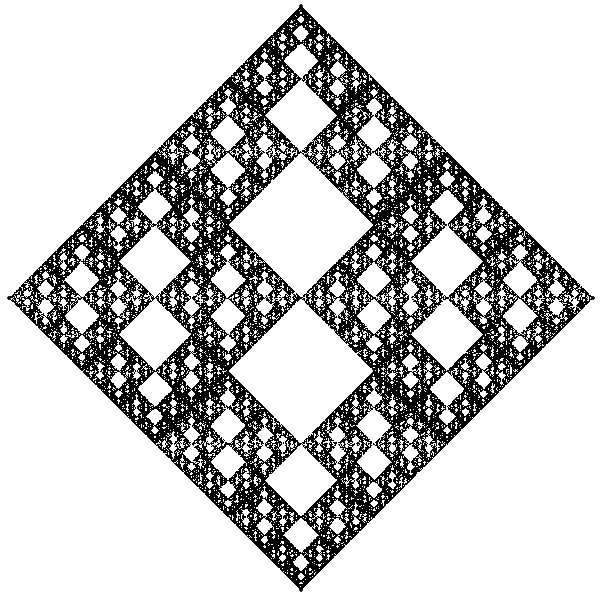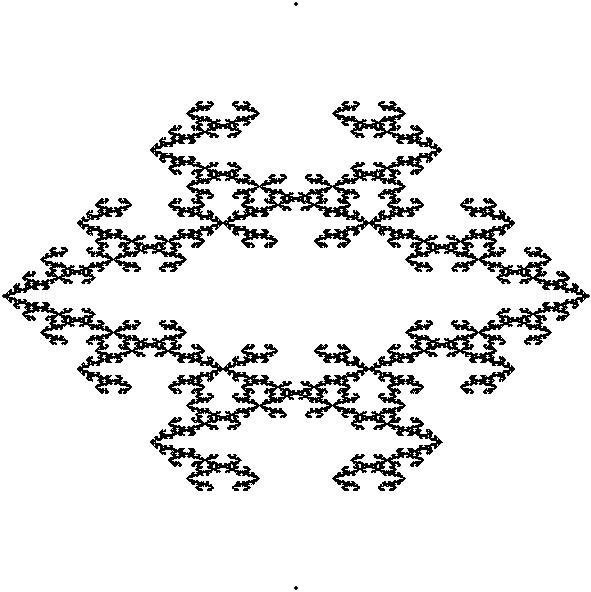# Chaos Game v2

I prepared a small demonstration of the Chaos game for some math teachers. First we used transparencies, markers, dice, and rulers, but humans are mistake-prone and slow.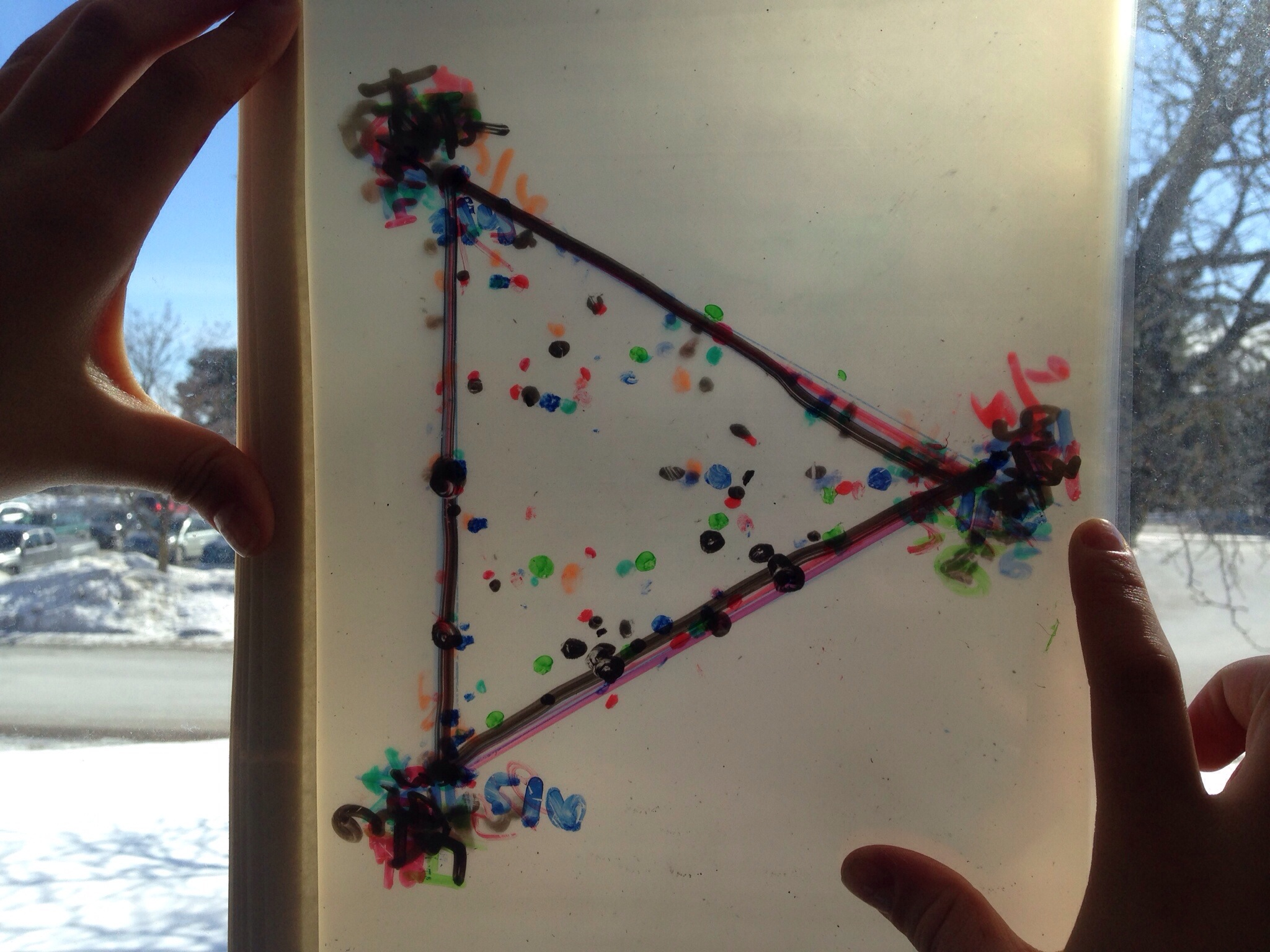## To The Computer!

I decided to experiment with the rules of the game to see where it’d go.

## Human Error

What happens when you bring in a random amount of error based on the distance traveled? Can you predict the result? The further the distance, the more error in the calculation.

Random Square

Random Hexagon

## Weighted Die

What happens when you use a weighted die? Can you tell which vertex is being chosen more often than the others?

Weighted Die Triangle

Weighted Die Hexagon

## Generalized Chaos Game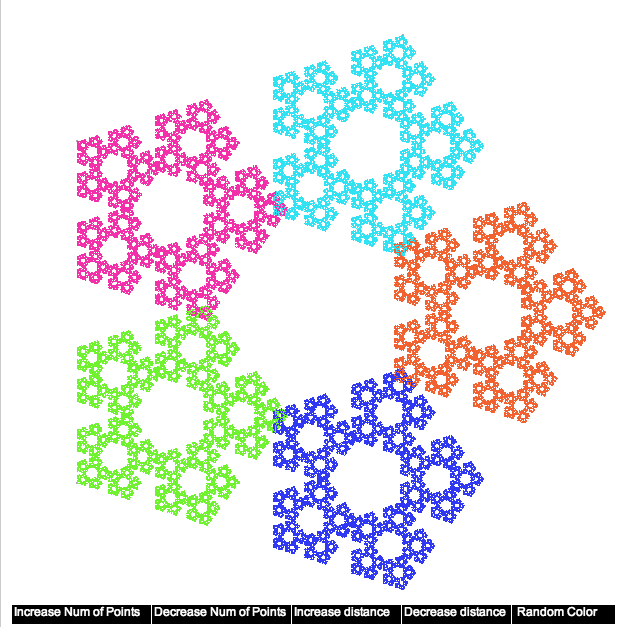By this time, I was getting frustrated at using different processing files just because I wanted to change the number of vertices. I had originally “cheated” to find the coordinates of each point by using a website to calculate the rectangular coordinates of a hexagon. I knew there must be an easier way, but last summer I was more focused on the creation of the images. I figured it out this time.

Here’s the code:

```//create points
float h1 = random(0.95);
for (float theta = 0; theta < TWO_PI; theta += TWO_PI / numOfPoints)
{
//graph the points using polar form (rotate theta), then convert back to rectangular
int xposition = int(radius * cos(theta))+width/2; //Add half width to move to 1st quad
int yposition = int(radius * sin(theta))+width/2;

h1 = h1 + 0.618033988749895; //Golden ratio!!
h1 = h1 % 1;

color c = color(h1,0.8,0.95);
}```

For those who are code-adverse, instead of trying to calculate the rectangular coordinates of a regular n-gon directly, I calculate the polar coordinates and then convert back to rectangular. Duh! Notice the theta going from 0 to TWO_PI, and going up by (TWO_PI / numOfPoints), and then going back to rectangular by using x = r*cos (theta) and y = r*sin(theta).

This code snippet also highlights a neat trick to make the random colors that were spread out nicely (idea from here). The problem is that using random to get a decimal from 0 to 1 doesn’t tend to spread the color values out, there are lots of repeat colors. But if you chose the first color randomly, and then rotate by the golden ratio (add the conjugate:  0.618033988749895), then you get colors that start off random, but are nicely spread out. Here’s an example of the problem. The top graph is twenty random numbers, and the bottom graph is one random number spread out to 20 points with help from the golden ratio.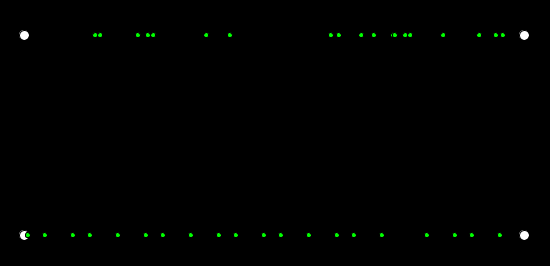Code.

## Some Interesting Results

Go to the generalized chaos game, and reduce the number of vertices to 4, and increase the distance by two clicks (60% of the way to the next random choice). You should get something like this: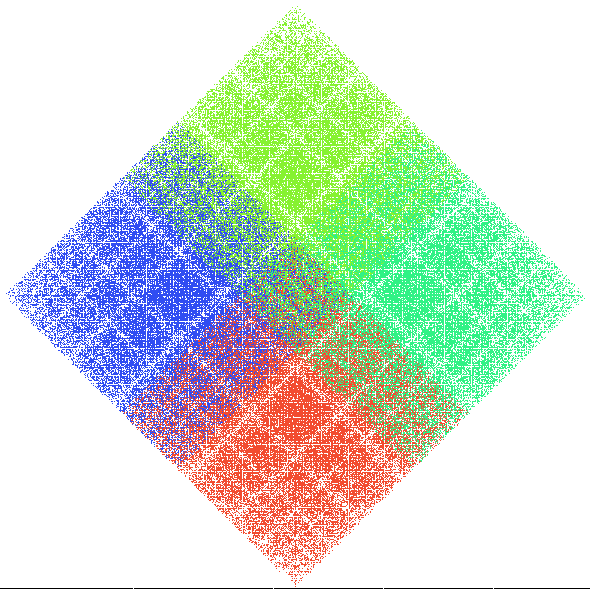Do the white lines occur at a geometric sequence? Why??

Now go to the hexagon, and increase the distance two clicks again, you should get something like this: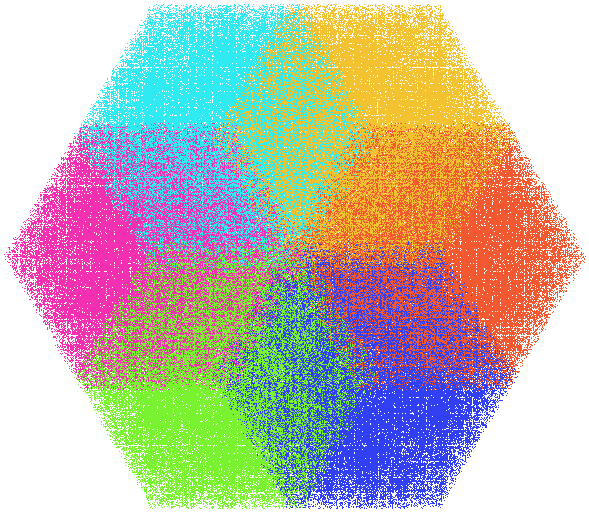What shapes are the overlapping colors (and the primary colors)? Why?

Lastly, some fun patterns happen when you continue to decrease the distance. Eventually, each point will actually move away from the randomly chosen point. Here are two such versions.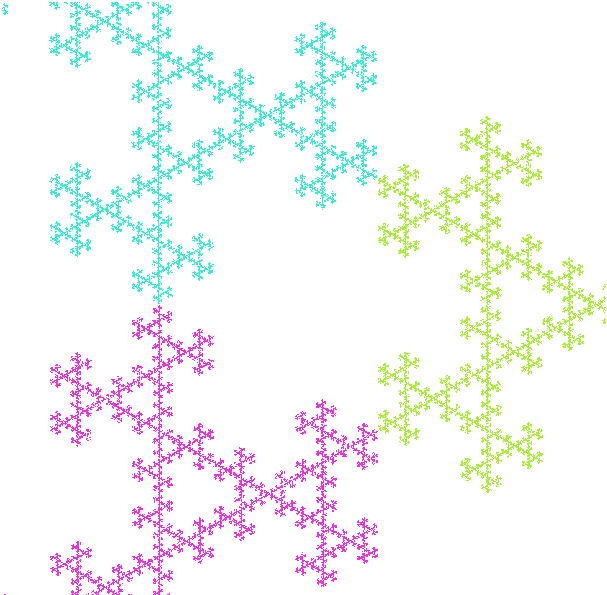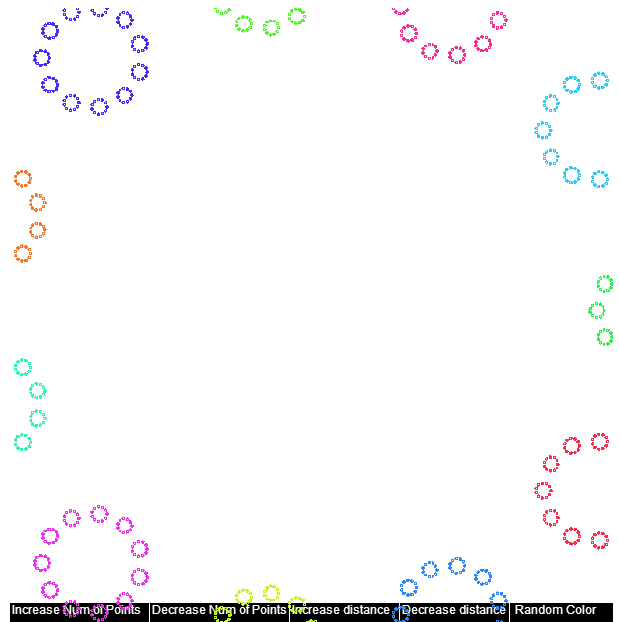## Happy Accidents

While trying to make the generalized chaos program, I ran into the following by accident: